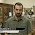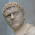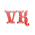## Thursday, 15 October 2015

### Mildly Interesting Math Finding: Areas between trivial zeros of the Riemann Zeta function.

One of the reasons I started this blog was to talk about things that I have discovered that are interesting enough to share but not interesting enough to publish. This is one of those things. It's something I found out about the Riemann Zeta function when I was bored and playing around with Maple. I apologize in advance because the LaTeX on this page looks awful.

The Riemann Zeta function, $\zeta(x)$ is the sum of every integer to the power of -x:

$\zeta(x)=\sum_{n=1}^{\infty}\frac{1}{n^x}=\frac{1}{1^x}+\frac{1}{2^x}+\frac{1}{3^x}...$

It is undefined at x=1, at x=2 it evaluates to $\pi^{2}/6$, and generally decreases over the positive numbers. When evaluated for complex numbers, using the necessary analytic continuation of the function, it occasionally equals zero. All known Zeta zeros of complex numbers have a real part of 1/2, and if there are no exceptions to this trend, it has profound implications for the distribution of prime numbers. This is known as the Riemann hypothesis, and its solution is worth a million dollars. Recently, there was a viral video about how $\zeta(-1)=1+2+3+4...=-1/12$, which isn't quite right.

What are less interesting than the non-trivial zeros are the trivial zeros: negative even numbers. Negative even numbers make the Zeta function equal zero because a re-writing of the equation for negative numbers includes a sine function with a period of two which has a phase of $\pi$ for negative integers.

One day I was plotting the Riemann Zeta function over negative numbers for some reason. It looks like this:The less popular strip of the Riemann Zeta function.
After some initial adjustment, the area subtended by the curve between each of the zeros gets bigger and bigger. In fact, after this graph truncates to the left, it starts getting too big to reasonably show on a graph.

Out of curiosity, I calculated the integrals of the curve between each zero (we'll call them G), and looked at it. This grows extremely rapidly, blowing the exponential function out of the water and surpassing $x^x$ at around the 70th interval.The numbers are too big for my regular graph-making program to handle.

I have a vague recollection of what I did with these numbers (I think it was 2010?), so I tried to re-create it when I thought of this exercise tonight. Let's look at the ratio of each interval to the one before it.

This is a pretty well-behaved function, and if you look at it on logarithmic axes you can squint a straight line into existence. This implies that the ratio is described by a power law, and if you do a naive power-law fit you get an exponent of like 1.97. That's almost quadratic! Performing a quadratic fit to this data gives the function:

$G(i)=0.101i^{2}+0.298i-0.036$

There is some uncertainty on the intercept term, but the index coefficients are pretty tight. The R-squared coefficient is 1, it is basically a perfect fit.

So, this tells us something:

$|\frac{\int_{2(i-2)}^{2(i-1)}\zeta(x)dx}{\int_{2(i-1)}^{2i}\zeta(x)dx}|=0.101i^{2}+0.298i-0.036$

Coupled with the initial value for i=1, $G_{1}=.011$, we can find the magnitude of each interval iteratively.
$\int_{2(i-1)}^{2i}\zeta(x)dx=(-1)^{i}0.011 \times \prod_{n=2}^{i}\left[0.101n^{2}+0.298n-0.036\right]$

Interestingly, this product has a closed form solutions in terms of gamma functions of the roots of the quadratic polynomial describing the ratio:

$\frac{{a}^{i}\Gamma \left( 1/2\,{\frac {2\,ia+b-\sqrt {{b}^{2}-4\,ac}}{a}} \right) \Gamma \left( 1/2\,{\frac {2\,ia+b+\sqrt {{b}^{2}-4\,ac}}{a} } \right)}{{a}^{2} \left( \Gamma \left( 1/2\,{\frac {4\,a+b-\sqrt {{b }^{2}-4\,ac}}{a}} \right) \right) \left( \Gamma \left( 1/2\,{ \frac {4\,a+b+\sqrt {{b}^{2}-4\,ac}}{a}} \right) \right) }$

I plugged in the fitting parameters for a, b, and c, but didn't have much success predicting the interval integrals; I could get within a factor of two for some of them. This does however explain the growth of this interval sizes: its an exponential times the square of a factorial, so it should grow roughly like $x^{x}x!$.

Taking in all this information, I don't really know what this means, whether it's obvious or interesting or if it can be derived or what. It's hard to search for trivial zeros of the Riemann Zeta function because I just get stuff on non-trivial zeros. I don't know if this quadratic ratio behaviour is unique to the Riemann Zeta function, or holds for any sufficiently complex function. I'm treating these numbers as empirical phenomena, and it would be cool to know if there are first-principles behind them. This was something I came across stumbling blindly through math land, so if anyone has any insight I'd be happy to read it.

1.What's wrong with the analytic extension to give -1/12?

1.Infinity isn't a twelfth! Generally there are issues with doing basic arithmetic with infinite quantities. Basically in some contexts you can substitute the infinite sum for the negative fraction, but they don't equal each other. I think this article plus the comments summarize it: http://physicsbuzz.physicscentral.com/2014/01/redux-does-1234-112-absolutely-not.html

2.So, if I interpret that right, it's the area under the curve once you throw out any parts that diverge. I've seen different solutions used, but I guess they get at the same point. I guess that number still has value for some, though?

3.Another way to look at it is that $\sum_{n=1}^{\infty} n^{-s}$ only defines a function for $s$ where $\Re s > 1$. Using the idea of analytic continuation, you can get a function that is meromorphic (analytic everywhere aside from some exceptions) that has the same values as $\sum_{n=1}^{\infty} n^{-s}$ does for $\Re s > 1$, but is also defined for $\Re s \leq 1$ as well (again, except for $s \neq 1$). This new function is $-\frac{1}{12}$ at $s = -1$, which if you plugged into $\sum_{n=1}^{\infty} n^{-s}$ would get you $1 + 2 + 3 + \cdots$, but that is a divergent sum, so it doesn't have a value.

2.Interesting the last step involving the Gamma functions.
If it is trivial, I have missed something. Do you have a reference for it?

1.The last step follows by writing the product over the quadratic terms into a product over two pairs of linear progressions, and then recognizing a product over a linear progression as a gamma ratio.

2.The last step follows by writing the product over the quadratic terms into a product over two pairs of linear progressions, and then recognizing a product over a linear progression as a gamma ratio.

3.The other commenter has some insight, I got it by entering into Maple!

4.Wolfram|Alpha has free computations of this type:

http://wolfr.am/7z-2R~a1

3.Ok thanks, I'll look for properties of products of linear progression. It may be trivial but I've never worked with it.
Good post and good blog, btw!!

1.4.I think I have an explanation for your observation, though your ratio might be flipped. Let $G_{i+1} = \left| \frac{\int_{2i}^{2(i+1)} \zeta(-s) \, ds}{\int_{2(i-1)}^{2i} \zeta(-s) \, ds} \right|$. By the functional equation, we have $\zeta(-s) = 2(2\pi)^{-(1+s)}\cos\left(\frac{\pi}{2}(1+s)\right)\Gamma(1+s)\zeta(1+s)$. Cancelling out the twos out front, we have $G_{i+1} = \left| \frac{\int_{2i}^{2(i+1)} (2\pi)^{-(1+s)}\cos\left(\frac{\pi}{2}(1+s)\right)\Gamma(1+s)\zeta(1+s) \, ds}{\int_{2(i-1)}^{2i} (2\pi)^{-(1+s)}\cos\left(\frac{\pi}{2}(1+s)\right)\Gamma(1+s)\zeta(1+s) \, ds} \right|$. By applying $t=s-2$ in the numerator, we end up with $G_{i+1} = \left| \frac{\int_{2(i-1)}^{2i} (2\pi)^{-(3+t)}\cos\left(\frac{\pi}{2}(3+t)\right)\Gamma(3+t)\zeta(3+t) \, dt}{\int_{2(i-1)}^{2i} (2\pi)^{-(1+s)}\cos\left(\frac{\pi}{2}(1+s)\right)\Gamma(1+s)\zeta(1+s) \, ds} \right|$, so the bounds of integration match. We can pull a $\frac{1}{(2\pi)^2}$ out of the numerator, apply $\cos(x+\pi)=-\cos(x)$, and use the identity $\Gamma(x+1)=x\Gamma(x)$ twice to get $G_{i+1} = \frac{1}{(2\pi)^2} \left| \frac{\int_{2(i-1)}^{2i} (2\pi)^{-(1+t)}\cos\left(\frac{\pi}{2}(1+t)\right)(2+t)(1+t)\Gamma(1+t)\zeta(3+t) \, dt}{\int_{2(i-1)}^{2i} (2\pi)^{-(1+s)}\cos\left(\frac{\pi}{2}(1+s)\right)\Gamma(1+s)\zeta(1+s) \, ds} \right|$. $\zeta(s)$ is fairly flat along the real axis as $s \to \infty$ , so we can make the following inequality without losing too much: $G_{i+1} \leq \frac{1}{(2\pi)^2} \left| \frac{\int_{2(i-1)}^{2i} (2\pi)^{-(1+t)}\cos\left(\frac{\pi}{2}(1+t)\right)(2+t)(1+t)\Gamma(1+t) \, dt}{\int_{2(i-1)}^{2i} (2\pi)^{-(1+s)}\cos\left(\frac{\pi}{2}(1+s)\right)\Gamma(1+s) \, ds} \right|$ (Note that we could have picked the upper bound of the integral to plug into our $\zeta$s instead to get an inequality in the other direction). Let's clean things up a bit by letting $M(u) = (2\pi)^{-(1+u)}\, \left|\cos\left(\frac{\pi}{2}(1+u)\right)\right| \,\Gamma(1+u)$. Taking advantage of the fact that our $\cos$ term is either entirely positive or entirely negative over the region of integration our inequality becomes $G_{i+1} \leq \frac{1}{(2\pi)^2} \frac{\int_{2(i-1)}^{2i} (t+2)(t+1)M(t) \,dt}{\int_{2(i-1)}^{2i} M(s) \,ds} = \frac{1}{(2\pi)^2} \left[ \frac{\int_{2(i-1)}^{2i} t^2 M(t) \,dt}{\int_{2(i-1)}^{2i} M(s) \,ds} + 3 \frac{\int_{2(i-1)}^{2i} t M(t) \,dt}{\int_{2(i-1)}^{2i} M(s) \,ds} +2 \right]$. Finally, since $M(u)$ is monotone and increasing over the range of integration, we'll use $\int_{a}^{b}t^nM(t)\,dt \leq b^n \int_a^b M(t)\,dt$ to finally get $G_{i+1} \leq \frac{1}{(2\pi)^2} \left[ 4i^2 + 6i + 2 \right]$. We could have instead pulled $\frac{\zeta(2i+3)}{\zeta(2i-1)}$ out of the integrals when we were dealing with the $\zeta$ factors earlier and had an inequality in the other direction, and with a bit more effort, showed that $G_i = \Theta(i^2)$, but I've already been hand wavy enough this comment. :D

1.Very interesting! If you evaluate the first term of your quadratic inequality, you get something quite close to my fit parameter (.101). The other ones are off by ~factor of two.